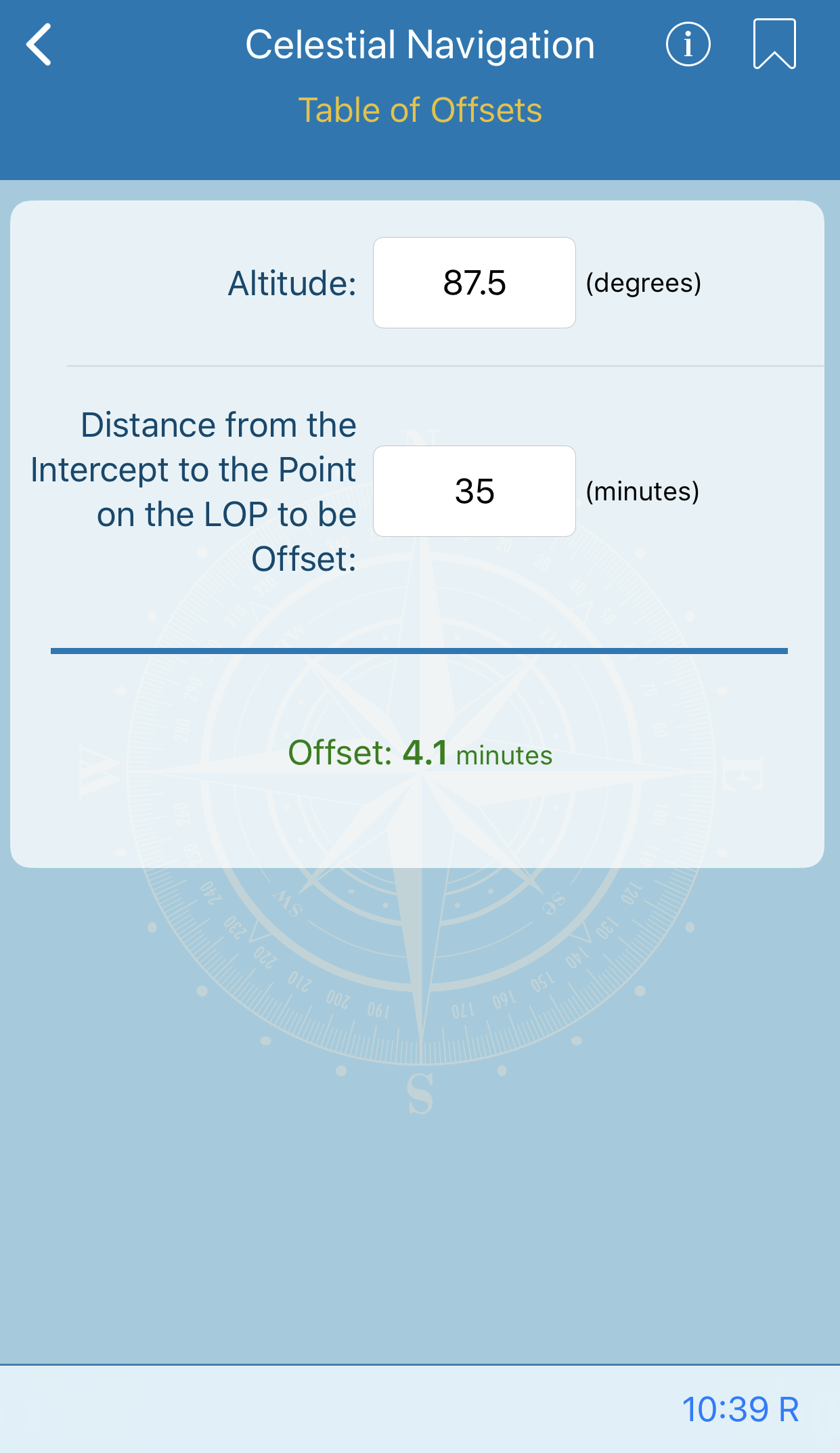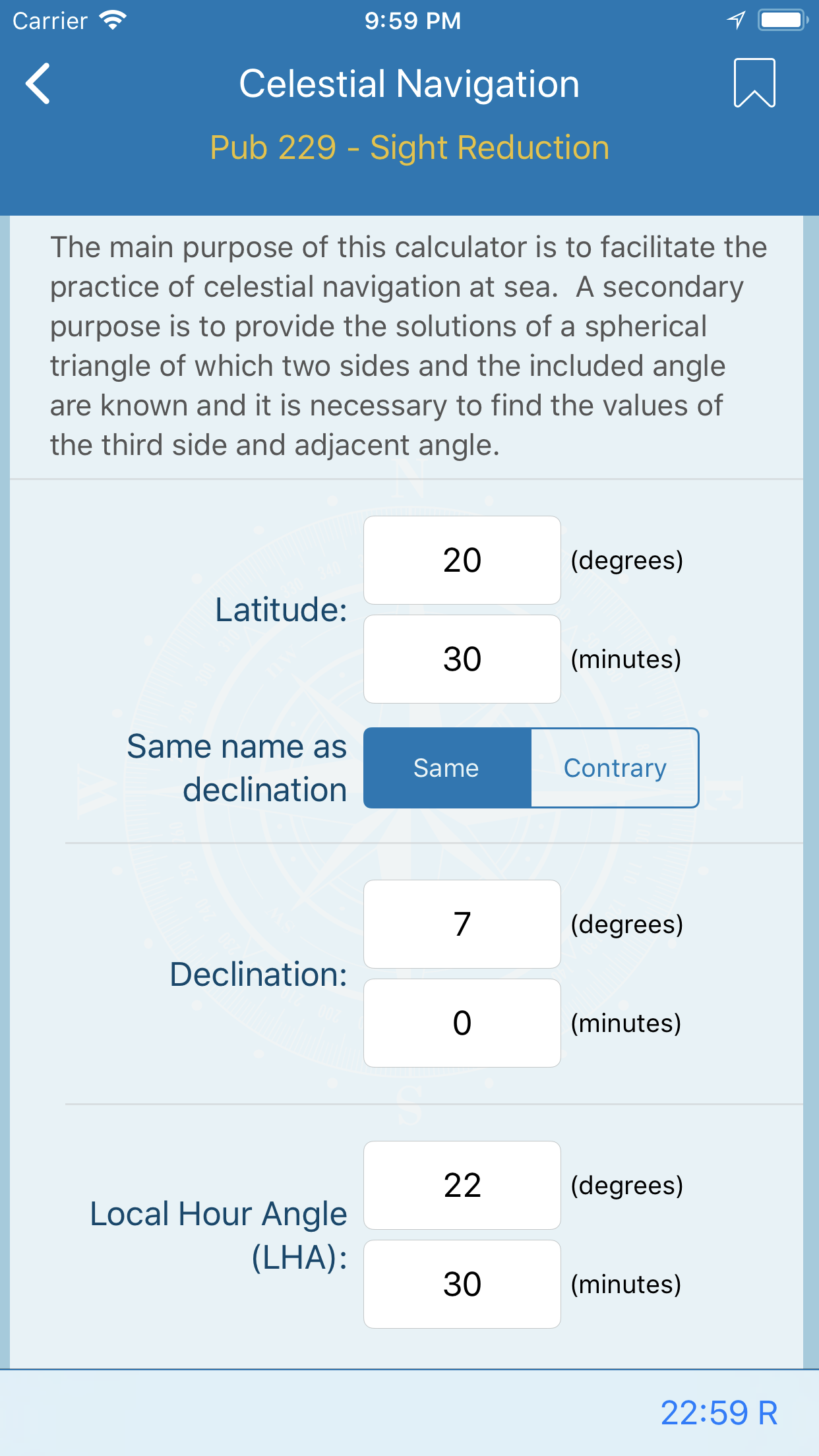free bootstrap templates## Compass Error from Amplitudes Observed on the Visible Horizon

This calculation contains a correction to be applied to the amplitude observed when the center of a ceslestial body is on the visible horizon, to obtain the corresponding amplitude when the center of the body is on the celestial horizon.## Altitude Correction for Air Temperature

This calculation provides a correction to be applied to the altitude of a celestial body when the air temperature varies from the 50° F used for determining mean refraction with the Nautical Almanac. For maximum accuracy, apply index correction and dip to sextant altitude first, obtaining apparent (rectified) altitude.## Table of Offsets

This calculation gives the corrections to the straight line of position (LOP) as drawn on a chart or plotting sheet to provide a closer approximation to the arc of the circle of equal altitude, a small circle of radius equal to the zenith distance.## Latitude and Longitude Factors

The latitude obtained by an ex-meridian sight is inaccurate if the longitude used in determining the meridian angle is incorrect. Similarly, the longitude obtained by solution of a time sight is inaccurate if the latitude used in the solution is incorrect, unless the celestial body is on the prime vertical.

This calculation gives the errors resulting from unit errors in the assumed values used in the computations.## Altitude Correction for Atmospheric Pressure

This calculation provides a correction to be applied to the altitude of a celestial body when the atmospheric pressure varies from the 29.83 inches (1010 millibars) used for determining mean refraction using the Nautical Almanac.## Altitude Factors & Change of Altitude

This calculation is used in solving reduction to the meridian (ex-meridian) problems.  It calculates the change in the altitude of a celestial body in one miute of time from meridian transit and can be applied as a correction to observed altitude to obtain the corresponding meridian altitude, adding for upper transit and subtracting for lower transit.## Change of Altitude in Given Time from Meridian Transit

This calculation is used in solving reduction to the meridian (ex-meridian) problems. It calculates the change in the altitude of a celestial body in one miute of time from meridian transit and can be applied as a correction to observed altitude to obtain the corresponding meridian altitude, adding for upper transit and subtracting for lower transit.## Pub 229 - Sight Reduction

Calculates the data in the Sight Reduction Tables for Marine Navigation (Pub. No. 229).

The main purpose of this calculator is to facilitate the practice of celestial navigation at sea. A secondary purpose is to provide the solutions of a spherical triangle of which two sides and the included angle are known and it is necessary to find the values of the third side and adjacent angle.

Furthur, you'll find a six-volume set of sight reduction tables in the reference section of the app.## Compass Error from Amplitudes Observed on the Celestial Horizon

This calculation lists amplitudes of celestial bodies at rising and setting. Enter with the declination of the body and the latitude of the observer. The value derived is the amplitude when the center of the body is on the celestial horizon. For the sun, this occurs when the lower limb is a little more than half a diameter above the visible horizon. For the moon it occurs when the upper limb is about on the horizon.## Meridian Angle and Altitude of a Body on the Prime Vertical Circle

The calculation is entered with declination of the celestial body and the latitude of the observer. Computed altitudes are given, with no allowance made for refraction, dip, parallax, etc. The calculated values apply to any celestial body, but values are not given for declination greater than 23° because the tabulated information is generally desired for the sun only.